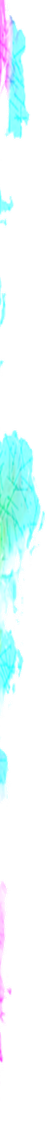### Line

To draw a line

• turned_in_notLine Related Tag
• `<line>`

##### <line>

Used to define a line, to draw it on svg you have to use additional attribute stroke

Syntax

``<line x1="point" y1="point" x2="point" y2="point" />``
• label_outlineLine Attributes
• x1 - Start point at x-axis
• y1 - Start point at y-axis
• x2 - End point at x-axis
• y2 - End point at y-axis
``````<svg height="100" width="200">
<line x1="10" y1="50" x2="180" y2="50" style="stroke:red;"/>
</svg>``````
Line

• Rectanglereceipt